Intermediate Algebra

# 4.4Solve Systems of Equations with Three Variables

Intermediate Algebra4.4 Solve Systems of Equations with Three Variables

### Learning Objectives

By the end of this section, you will be able to:
• Determine whether an ordered triple is a solution of a system of three linear equations with three variables
• Solve a system of linear equations with three variables
• Solve applications using systems of linear equations with three variables

### Be Prepared 4.4

Before you get started, take this readiness quiz.

1. Evaluate $5x−2y+3z5x−2y+3z$ when $x=−2,x=−2,$ $y=−4,y=−4,$ and $z=3.z=3.$
If you missed this problem, review Example 1.21.
2. Classify the equations as a conditional equation, an identity, or a contradiction and then state the solution. ${−2x+y=−11x+3y=9.{−2x+y=−11x+3y=9.$
If you missed this problem, review Example 2.6.
3. Classify the equations as a conditional equation, an identity, or a contradiction and then state the solution. ${7x+8y=43x−5y=27.{7x+8y=43x−5y=27.$
If you missed this problem, review Example 2.8.

### Determine Whether an Ordered Triple is a Solution of a System of Three Linear Equations with Three Variables

In this section, we will extend our work of solving a system of linear equations. So far we have worked with systems of equations with two equations and two variables. Now we will work with systems of three equations with three variables. But first let's review what we already know about solving equations and systems involving up to two variables.

We learned earlier that the graph of a linear equation, $ax+by=c,ax+by=c,$ is a line. Each point on the line, an ordered pair $(x,y),(x,y),$ is a solution to the equation. For a system of two equations with two variables, we graph two lines. Then we can see that all the points that are solutions to each equation form a line. And, by finding what the lines have in common, we’ll find the solution to the system.

Most linear equations in one variable have one solution, but we saw that some equations, called contradictions, have no solutions and for other equations, called identities, all numbers are solutions

We know when we solve a system of two linear equations represented by a graph of two lines in the same plane, there are three possible cases, as shown.Similarly, for a linear equation with three variables $ax+by+cz=d,ax+by+cz=d,$ every solution to the equation is an ordered triple, $(x,y,z)(x,y,z)$, that makes the equation true.

### Linear Equation in Three Variables

A linear equation with three variables, where a, b, c, and d are real numbers and a, b, and c are not all 0, is of the form

$ax+by+cz=dax+by+cz=d$

Every solution to the equation is an ordered triple, $(x,y,z)(x,y,z)$ that makes the equation true.

All the points that are solutions to one equation form a plane in three-dimensional space. And, by finding what the planes have in common, we’ll find the solution to the system.

When we solve a system of three linear equations represented by a graph of three planes in space, there are three possible cases.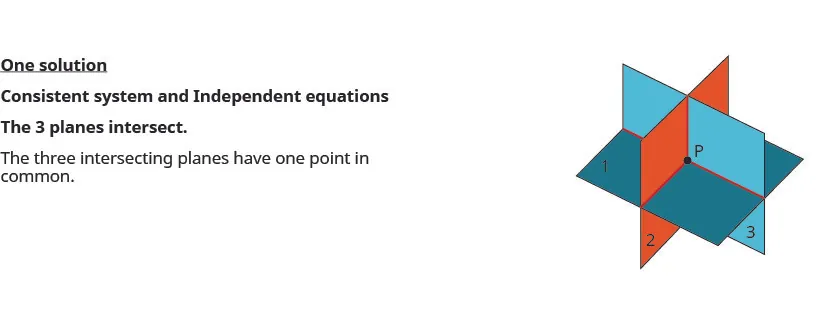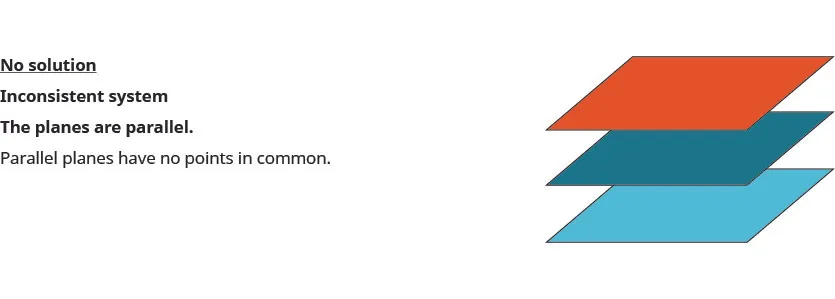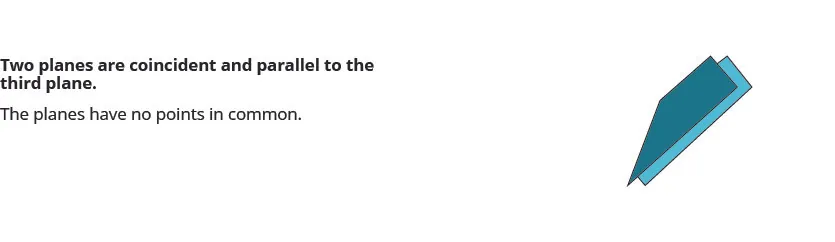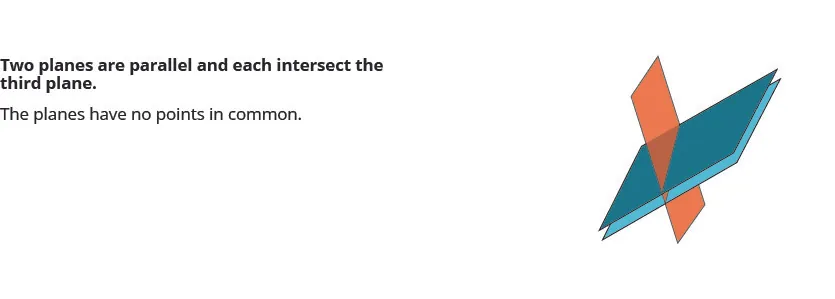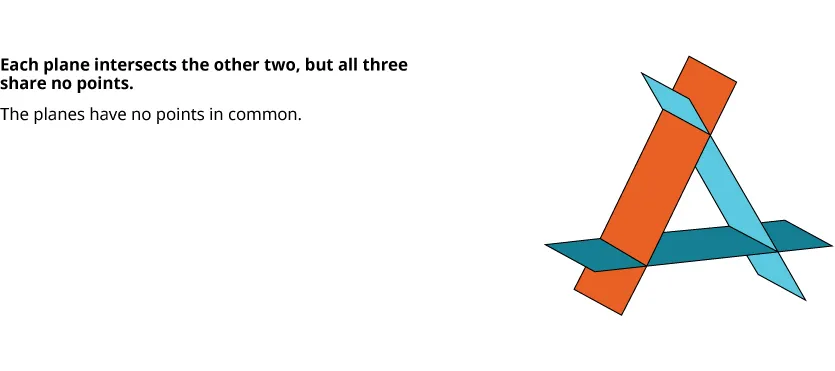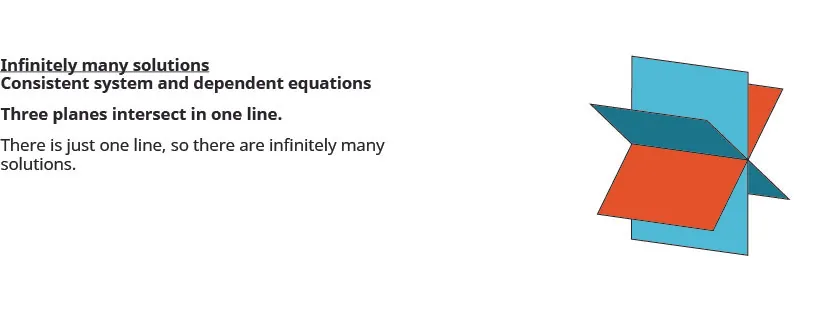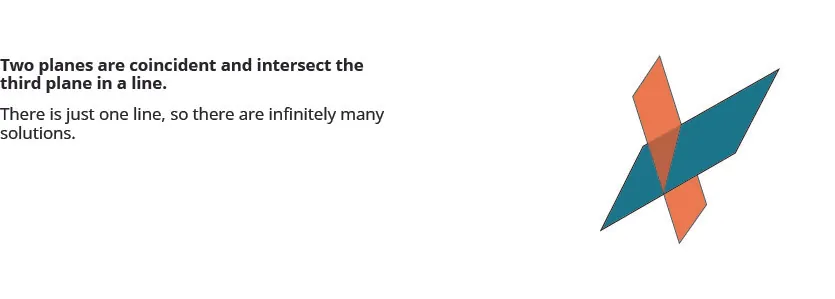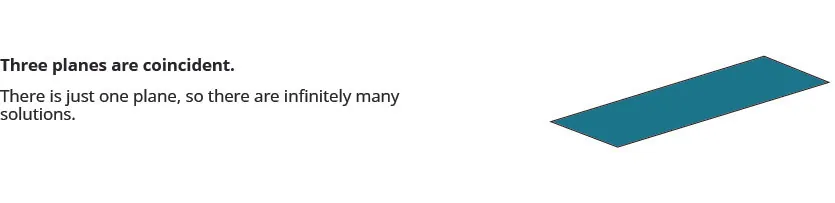To solve a system of three linear equations, we want to find the values of the variables that are solutions to all three equations. In other words, we are looking for the ordered triple $(x,y,z)(x,y,z)$ that makes all three equations true. These are called the solutions of the system of three linear equations with three variables.

### Solutions of a System of Linear Equations with Three Variables

Solutions of a system of equations are the values of the variables that make all the equations true. A solution is represented by an ordered triple $(x,y,z).(x,y,z).$

To determine if an ordered triple is a solution to a system of three equations, we substitute the values of the variables into each equation. If the ordered triple makes all three equations true, it is a solution to the system.

### Example 4.31

Determine whether the ordered triple is a solution to the system: ${x−y+z=22x−y−z=−62x+2y+z=−3.{x−y+z=22x−y−z=−62x+2y+z=−3.$

$(−2,−1,3)(−2,−1,3)$ $(−4,−3,4)(−4,−3,4)$

### Try It 4.61

Determine whether the ordered triple is a solution to the system: ${3x+y+z=2x+2y+z=−33x+y+2z=4.{3x+y+z=2x+2y+z=−33x+y+2z=4.$

$(1,−3,2)(1,−3,2)$ $(4,−1,−5)(4,−1,−5)$

### Try It 4.62

Determine whether the ordered triple is a solution to the system: ${x−3y+z=−5−3x−y−z=12x−2y+3z=1.{x−3y+z=−5−3x−y−z=12x−2y+3z=1.$

$(2,−2,3)(2,−2,3)$ $(−2,2,3)(−2,2,3)$

### Solve a System of Linear Equations with Three Variables

To solve a system of linear equations with three variables, we basically use the same techniques we used with systems that had two variables. We start with two pairs of equations and in each pair we eliminate the same variable. This will then give us a system of equations with only two variables and then we know how to solve that system!

Next, we use the values of the two variables we just found to go back to the original equation and find the third variable. We write our answer as an ordered triple and then check our results.

### Example 4.32

#### How to Solve a System of Equations With Three Variables by Elimination

Solve the system by elimination: ${x−2y+z=32x+y+z=43x+4y+3z=−1.{x−2y+z=32x+y+z=43x+4y+3z=−1.$

### Try It 4.63

Solve the system by elimination: ${3x+y−z=22x−3y−2z=14x−y−3z=0.{3x+y−z=22x−3y−2z=14x−y−3z=0.$

### Try It 4.64

Solve the system by elimination: ${4x+y+z=−1−2x−2y+z=22x+3y−z=1.{4x+y+z=−1−2x−2y+z=22x+3y−z=1.$

The steps are summarized here.

### How To

#### Solve a system of linear equations with three variables.

1. Step 1.
Write the equations in standard form
• If any coefficients are fractions, clear them.
2. Step 2.
Eliminate the same variable from two equations.
• Decide which variable you will eliminate.
• Work with a pair of equations to eliminate the chosen variable.
• Multiply one or both equations so that the coefficients of that variable are opposites.
• Add the equations resulting from Step 2 to eliminate one variable
3. Step 3. Repeat Step 2 using two other equations and eliminate the same variable as in Step 2.
4. Step 4. The two new equations form a system of two equations with two variables. Solve this system.
5. Step 5. Use the values of the two variables found in Step 4 to find the third variable.
6. Step 6. Write the solution as an ordered triple.
7. Step 7. Check that the ordered triple is a solution to all three original equations.

### Example 4.33

Solve: ${3x−4z=03y+2z=−32x+3y=−5.{3x−4z=03y+2z=−32x+3y=−5.$

### Try It 4.65

Solve: ${3x−4z=−12y+3z=22x+3y=6.{3x−4z=−12y+3z=22x+3y=6.$

### Try It 4.66

Solve: ${4x−3z=−53y+2z=73x+4y=6.{4x−3z=−53y+2z=73x+4y=6.$

When we solve a system and end up with no variables and a false statement, we know there are no solutions and that the system is inconsistent. The next example shows a system of equations that is inconsistent.

### Example 4.34

Solve the system of equations: ${x+2y−3z=−1x−3y+z=12x−y−2z=2.{x+2y−3z=−1x−3y+z=12x−y−2z=2.$

### Try It 4.67

Solve the system of equations: ${x+2y+6z=5−x+y−2z=3x−4y−2z=1.{x+2y+6z=5−x+y−2z=3x−4y−2z=1.$

### Try It 4.68

Solve the system of equations: ${2x−2y+3z=64x−3y+2z=0−2x+3y−7z=1.{2x−2y+3z=64x−3y+2z=0−2x+3y−7z=1.$

When we solve a system and end up with no variables but a true statement, we know there are infinitely many solutions. The system is consistent with dependent equations. Our solution will show how two of the variables depend on the third.

### Example 4.35

Solve the system of equations: ${x+2y−z=12x+7y+4z=11x+3y+z=4.{x+2y−z=12x+7y+4z=11x+3y+z=4.$

### Try It 4.69

Solve the system by equations: ${x+y−z=02x+4y−2z=63x+6y−3z=9.{x+y−z=02x+4y−2z=63x+6y−3z=9.$

### Try It 4.70

Solve the system by equations: ${x−y−z=1−x+2y−3z=−43x−2y−7z=0.{x−y−z=1−x+2y−3z=−43x−2y−7z=0.$

### Solve Applications using Systems of Linear Equations with Three Variables

Applications that are modeled by a systems of equations can be solved using the same techniques we used to solve the systems. Many of the application are just extensions to three variables of the types we have solved earlier.

### Example 4.36

The community college theater department sold three kinds of tickets to its latest play production. The adult tickets sold for $15, the student tickets for$10 and the child tickets for $8. The theater department was thrilled to have sold 250 tickets and brought in$2,825 in one night. The number of student tickets sold is twice the number of adult tickets sold. How many of each type did the department sell?

### Try It 4.71

The community college fine arts department sold three kinds of tickets to its latest dance presentation. The adult tickets sold for $20, the student tickets for$12 and the child tickets for $10.The fine arts department was thrilled to have sold 350 tickets and brought in$4,650 in one night. The number of child tickets sold is the same as the number of adult tickets sold. How many of each type did the department sell?

### Try It 4.72

The community college soccer team sold three kinds of tickets to its latest game. The adult tickets sold for $10, the student tickets for$8 and the child tickets for $5. The soccer team was thrilled to have sold 600 tickets and brought in$4,900 for one game. The number of adult tickets is twice the number of child tickets. How many of each type did the soccer team sell?

### Media

Access this online resource for additional instruction and practice with solving a linear system in three variables with no or infinite solutions.

### Section 4.4 Exercises

#### Practice Makes Perfect

Determine Whether an Ordered Triple is a Solution of a System of Three Linear Equations with Three Variables

In the following exercises, determine whether the ordered triple is a solution to the system.

162.

${ 2 x − 6 y + z = 3 3 x − 4 y − 3 z = 2 2 x + 3 y − 2 z = 3 { 2 x − 6 y + z = 3 3 x − 4 y − 3 z = 2 2 x + 3 y − 2 z = 3$

$(3,1,3)(3,1,3)$ $(4,3,7)(4,3,7)$

163.

${ − 3 x + y + z = −4 − x + 2 y − 2 z = 1 2 x − y − z = −1 { − 3 x + y + z = −4 − x + 2 y − 2 z = 1 2 x − y − z = −1$

$(−5,−7,4)(−5,−7,4)$ $(5,7,4)(5,7,4)$

164.

${ y − 10 z = −8 2 x − y = 2 x − 5 z = 3 { y − 10 z = −8 2 x − y = 2 x − 5 z = 3$

$(7,12,2)(7,12,2)$ $(2,2,1)(2,2,1)$

165.

${ x + 3 y − z = 15 y = 2 3 x − 2 x − 3 y + z = −2 { x + 3 y − z = 15 y = 2 3 x − 2 x − 3 y + z = −2$

$(−6,5,12)(−6,5,12)$ $(5,43,−3)(5,43,−3)$

Solve a System of Linear Equations with Three Variables

In the following exercises, solve the system of equations.

166.

${ 5 x + 2 y + z = 5 − 3 x − y + 2 z = 6 2 x + 3 y − 3 z = 5 { 5 x + 2 y + z = 5 − 3 x − y + 2 z = 6 2 x + 3 y − 3 z = 5$

167.

${ 6 x − 5 y + 2 z = 3 2 x + y − 4 z = 5 3 x − 3 y + z = −1 { 6 x − 5 y + 2 z = 3 2 x + y − 4 z = 5 3 x − 3 y + z = −1$

168.

${ 2 x − 5 y + 3 z = 8 3 x − y + 4 z = 7 x + 3 y + 2 z = −3 { 2 x − 5 y + 3 z = 8 3 x − y + 4 z = 7 x + 3 y + 2 z = −3$

169.

${ 5 x − 3 y + 2 z = −5 2 x − y − z = 4 3 x − 2 y + 2 z = −7 { 5 x − 3 y + 2 z = −5 2 x − y − z = 4 3 x − 2 y + 2 z = −7$

170.

${ 3 x − 5 y + 4 z = 5 5 x + 2 y + z = 0 2 x + 3 y − 2 z = 3 { 3 x − 5 y + 4 z = 5 5 x + 2 y + z = 0 2 x + 3 y − 2 z = 3$

171.

${ 4 x − 3 y + z = 7 2 x − 5 y − 4 z = 3 3 x − 2 y − 2 z = −7 { 4 x − 3 y + z = 7 2 x − 5 y − 4 z = 3 3 x − 2 y − 2 z = −7$

172.

${ 3 x + 8 y + 2 z = −5 2 x + 5 y − 3 z = 0 x + 2 y − 2 z = −1 { 3 x + 8 y + 2 z = −5 2 x + 5 y − 3 z = 0 x + 2 y − 2 z = −1$

173.

${ 11 x + 9 y + 2 z = −9 7 x + 5 y + 3 z = −7 4 x + 3 y + z = −3 { 11 x + 9 y + 2 z = −9 7 x + 5 y + 3 z = −7 4 x + 3 y + z = −3$

174.

${ 1 3 x − y − z = 1 x + 5 2 y + z = −2 2 x + 2 y + 1 2 z = −4 { 1 3 x − y − z = 1 x + 5 2 y + z = −2 2 x + 2 y + 1 2 z = −4$

175.

${ x + 1 2 y + 1 2 z = 0 1 5 x − 1 5 y + z = 0 1 3 x − 1 3 y + 2 z = −1 { x + 1 2 y + 1 2 z = 0 1 5 x − 1 5 y + z = 0 1 3 x − 1 3 y + 2 z = −1$

176.

${ x + 1 3 y − 2 z = −1 1 3 x + y + 1 2 z = 0 1 2 x + 1 3 y − 1 2 z = −1 { x + 1 3 y − 2 z = −1 1 3 x + y + 1 2 z = 0 1 2 x + 1 3 y − 1 2 z = −1$

177.

${ 1 3 x − y + 1 2 z = 4 2 3 x + 5 2 y − 4 z = 0 x − 1 2 y + 3 2 z = 2 { 1 3 x − y + 1 2 z = 4 2 3 x + 5 2 y − 4 z = 0 x − 1 2 y + 3 2 z = 2$

178.

${ x + 2 z = 0 4 y + 3 z = −2 2 x − 5 y = 3 { x + 2 z = 0 4 y + 3 z = −2 2 x − 5 y = 3$

179.

${ 2 x + 5 y = 4 3 y − z = 3 4 x + 3 z = −3 { 2 x + 5 y = 4 3 y − z = 3 4 x + 3 z = −3$

180.

${ 2 y + 3 z = −1 5 x + 3 y = −6 7 x + z = 1 { 2 y + 3 z = −1 5 x + 3 y = −6 7 x + z = 1$

181.

${ 3 x − z = −3 5 y + 2 z = −6 4 x + 3 y = −8 { 3 x − z = −3 5 y + 2 z = −6 4 x + 3 y = −8$

182.

${ 4 x − 3 y + 2 z = 0 − 2 x + 3 y − 7 z = 1 2 x − 2 y + 3 z = 6 { 4 x − 3 y + 2 z = 0 − 2 x + 3 y − 7 z = 1 2 x − 2 y + 3 z = 6$

183.

${ x − 2 y + 2 z = 1 − 2 x + y − z = 2 x − y + z = 5 { x − 2 y + 2 z = 1 − 2 x + y − z = 2 x − y + z = 5$

184.

${ 2 x + 3 y + z = 12 x + y + z = 9 3 x + 4 y + 2 z = 20 { 2 x + 3 y + z = 12 x + y + z = 9 3 x + 4 y + 2 z = 20$

185.

${ x + 4 y + z = −8 4 x − y + 3 z = 9 2 x + 7 y + z = 0 { x + 4 y + z = −8 4 x − y + 3 z = 9 2 x + 7 y + z = 0$

186.

${ x + 2 y + z = 4 x + y − 2 z = 3 − 2 x − 3 y + z = −7 { x + 2 y + z = 4 x + y − 2 z = 3 − 2 x − 3 y + z = −7$

187.

${ x + y − 2 z = 3 − 2 x − 3 y + z = −7 x + 2 y + z = 4 { x + y − 2 z = 3 − 2 x − 3 y + z = −7 x + 2 y + z = 4$

188.

${ x + y − 3 z = −1 y − z = 0 − x + 2 y = 1 { x + y − 3 z = −1 y − z = 0 − x + 2 y = 1$

189.

${ x − 2 y + 3 z = 1 x + y − 3 z = 7 3 x − 4 y + 5 z = 7 { x − 2 y + 3 z = 1 x + y − 3 z = 7 3 x − 4 y + 5 z = 7$

Solve Applications using Systems of Linear Equations with Three Variables

In the following exercises, solve the given problem.

190.

The sum of the measures of the angles of a triangle is 180. The sum of the measures of the second and third angles is twice the measure of the first angle. The third angle is twelve more than the second. Find the measures of the three angles.

191.

The sum of the measures of the angles of a triangle is 180. The sum of the measures of the second and third angles is three times the measure of the first angle. The third angle is fifteen more than the second. Find the measures of the three angles.

192.

After watching a major musical production at the theater, the patrons can purchase souvenirs. If a family purchases 4 t-shirts, the video, and 1 stuffed animal, their total is $135. A couple buys 2 t-shirts, the video, and 3 stuffed animals for their nieces and spends$115. Another couple buys 2 t-shirts, the video, and 1 stuffed animal and their total is $85. What is the cost of each item? 193. The church youth group is selling snacks to raise money to attend their convention. Amy sold 2 pounds of candy, 3 boxes of cookies and 1 can of popcorn for a total sales of$65. Brian sold 4 pounds of candy, 6 boxes of cookies and 3 cans of popcorn for a total sales of $140. Paulina sold 8 pounds of candy, 8 boxes of cookies and 5 cans of popcorn for a total sales of$250. What is the cost of each item?

#### Writing Exercises

194.

In your own words explain the steps to solve a system of linear equations with three variables by elimination.

195.

How can you tell when a system of three linear equations with three variables has no solution? Infinitely many solutions?

#### Self Check

After completing the exercises, use this checklist to evaluate your mastery of the objectives of this section.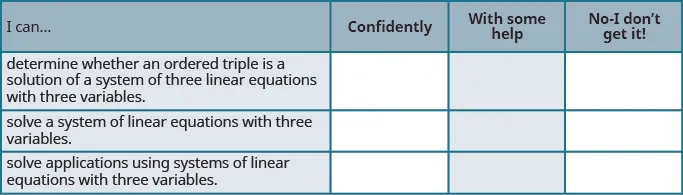On a scale of 1-10, how would you rate your mastery of this section in light of your responses on the checklist? How can you improve this?

Order a print copy

As an Amazon Associate we earn from qualifying purchases.